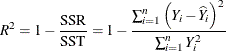#### Analysis of Variance

The identity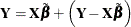holds for all vectors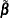, but only for the least squares solution is the residual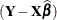orthogonal to the predicted value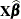. Because of this orthogonality, the additive identity holds not only for the vectors themselves, but also for their lengths (Pythagorean theorem):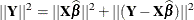Note that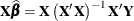=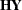and note that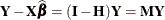. The matrices
and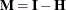play an important role in the theory of linear models and in statistical computations. Both are projection matrices—that is, they are symmetric and idempotent. (An idempotent matrix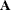is a square matrix that satisfies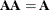. The eigenvalues of an idempotent matrix take on the values 1 and 0 only.) The matrixprojects onto the subspace of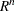that is spanned by the columns of. The matrix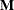projects onto the orthogonal complement of that space. Because of these properties you have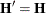,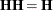,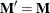,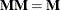,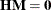.

The Pythagorean relationship now can be written in terms ofandas follows: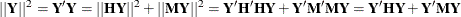If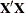is deficient in rank and a generalized inverse is used to solve the normal equations, then you work instead with the projection matrices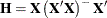. Note that ifis a generalized inverse of, then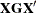, and hence alsoand, are invariant to the choice of.

The matrixis sometimes referred to as the hat matrix because when you premultiply the vector of observations with, you produce the fitted values, which are commonly denoted by placing a hat over the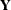vector,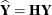.

The term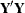is the uncorrected total sum of squares (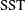) of the linear model,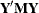is the error (residual) sum of squares (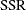), and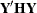is the uncorrected model sum of squares. This leads to the analysis of variance table shown in Table 3.2.

Table 3.2: Analysis of Variance with Uncorrected Sums of Squares

Source

df

Sum of Squares

Model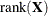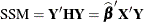Residual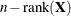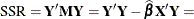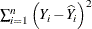Uncorr. Total

n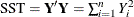When the model contains an intercept term, then the analysis of variance is usually corrected for the mean, as shown in Table 3.3.

Table 3.3: Analysis of Variance with Corrected Sums of Squares

Source

df

Sum of Squares

Model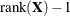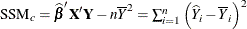ResidualCorrected Total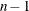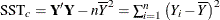The coefficient of determination, also called the R-square statistic, measures the proportion of the total variation explained by the linear model. In models with intercept, it is defined as the ratio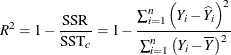In models without intercept, the R-square statistic is a ratio of the uncorrected sums of squares